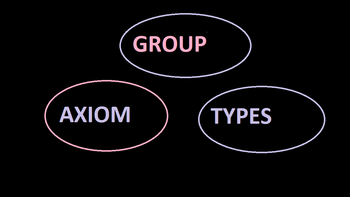# Group ,its axiom and typesGroup:

﻿An algebraic structure (G,*) , where G is a non- empty set with an operation '*' defined on it, is said to be a group  , if the operation * satisfies the following axioms.

• Closure axiom : G is closed under the operation *,  i.e. a*b belongs to G for all a,b belonging to G.

• Associative axiom: The binary operation * is associative .  i.e . (a*b)*c=a*(b*c) for  each a,b,c belongs to G.

• Identity axiom: There exists an element 'e' belonging to G, such that a*e=a=e*a, for all 'a' belonging to G

Inverse axiom: Each element of G possesses inverse , i.e. for each element 'a' belonging to G, there exists an element 'b' belonging to G, such that a*b=e=b*a, The element 'b' is then called the inverse of 'a' with respect to "*" and we write b= a^-1. Thus  a^-1 is an element of G such that a*a-1=e=a^-1*a.

Types:

1. ﻿Finite and infinite group:  A group may contain a finite or an infinite number of elements . It is said to be finite or infinite according as the number of elements is finite or infinite .The number of elements  in a group is often called the order of the group. It is denoted by |G| or o(G).

2.  Trivial group: A group consisting of only one element is called a trivial group.

3. Abelian group: A group (G,*) is said to be an abelian group if a*b=b*a for all a,b belonging to G.